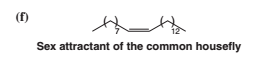# Problem: Classify each of the following compounds as an alkane, alkene, alkyne, alcohol, aldehyde, amine, and so forth.

###### Problem Details

Classify each of the following compounds as an alkane, alkene, alkyne, alcohol, aldehyde, amine, and so forth.What scientific concept do you need to know in order to solve this problem?

Our tutors have indicated that to solve this problem you will need to apply the Functional Groups concept. You can view video lessons to learn Functional Groups. Or if you need more Functional Groups practice, you can also practice Functional Groups practice problems.

What is the difficulty of this problem?

Our tutors rated the difficulty ofClassify each of the following compounds as an alkane, alken...as low difficulty.

How long does this problem take to solve?

Our expert Organic tutor, Chris took 1 minute and 8 seconds to solve this problem. You can follow their steps in the video explanation above.

What textbook is this problem found in?

Our data indicates that this problem or a close variation was asked in Organic Chemistry - Solomons 10th Edition. You can also practice Organic Chemistry - Solomons 10th Edition practice problems.